# Safe prime has plus or minus two as a primitive root

## Statement

Supposeis a safe prime, i.e.,is an odd prime such that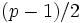is also prime (this is equivalent to saying thatis a Sophie Germain prime).

Then:

• If,is a primitive root modulo.
• If, then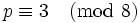, andis a primitive root modulo.
• If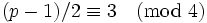, then, andis a primitive root modulo.

## Facts used

1. Quadratic nonresidue that is not minus one is primitive root for safe prime
2. Congruence condition for minus one to be a quadratic residue
3. Congruence condition for two to be a quadratic residue: For an odd prime,is a quadratic residue moduloiff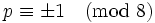, and a nonresidue iff.

## Proof

Given: A safe prime.

To prove: Ifor, thenis a primitive root modulo. If, thenis a primitive root modulo.

Proof: Since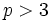, neithernoris congruent tomodulo. Thus, by fact (1), it suffices to show in either case that(or) is a quadratic nonresidue modulo.

The casesandare settled by fact (3).

In the case,. By facts (2) and (3),is a quadratic residue moduloandis a quadratic nonresidue modulo. Thus,is a quadratic nonresidue modulo, completing the proof.